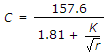# Civil Engineering - Waste Water Engineering

Exercise : Waste Water Engineering - Section 7
1.
In areas where light rains are uniformly distributed throughout the year, the type of sewerage system to be adopted is
separate system
combined system
partially combined system
none of these.
Explanation:
No answer description is available. Let's discuss.

2.
For detecting the nitrates in sewage, the colour may be developed by adding
Pottassium permanganate
Sulphuric acid and napthamine
Phenol-di-sulphuric acid and pottassium hydroxide
None of these.
Explanation:
No answer description is available. Let's discuss.

3.
The value of Chezy's constantis used in
Chezy's formula
Bazin's formula
Kutter's formula
Manning's formula.
Explanation:
No answer description is available. Let's discuss.

4.
The formula which accepts the value of rugosity coefficient n = 0.012 to be used in Manning's formula, is given by
Bazin
Crimp and Bruge
William-Hazen
Kutter.